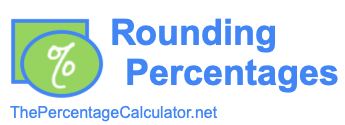Rounding PercentagesThis tool allows you to round any percentage you enter to however many decimals you want.

Simply enter the percentage and how many decimals you want below and then press "Round %".

Percentage:

Decimals:

Rounded Percentage:

Notes
When rounding percentages, we rounded up or down to the nearest number of decimals you wanted.

We rounded up if the next decimal was five or above, and down if it was four or less.